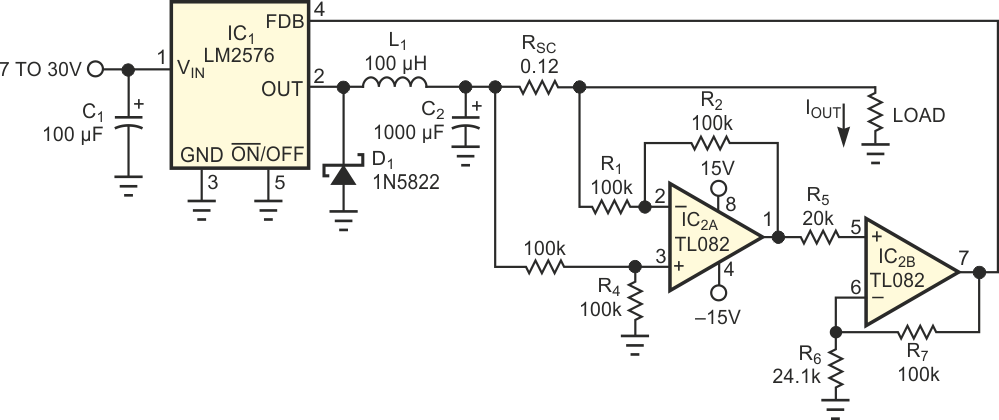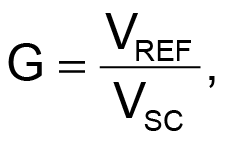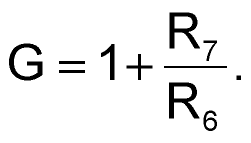# Switching regulator forms constant-current source

## Texas Instruments LM2576 TL082

Stefan Strozecki

EDN

Many applications require current sources rather than voltage sources. When you need a high-current source, using a linear regulator is inadvisable, because of the high power dissipation in the series resistor. To solve the wasted-power problem, you can use a switch-mode regulator. The circuit of Figure 1 uses IC1, an LM2576 adjustable regulator. It needs only a few external elements and has an adjustable sensing input, which you use for controlling the output current. Resistor RSC is a current sensor. IC2A, one-half of a TL082 op amp, operates as a difference amplifier. When R1 = R2 = R3 = R4, the output voltage is proportional to the current flowing in RSC. Good common-mode rejection and a wide common-mode voltage range are important, because the amplifier works with large, changing common-mode signals.Figure 1. A switching voltage regulator forms a good basis for a constant-current source.

The second half of the TL082 op amp, IC2B, operates as a noninverting amplifier. The required gain depends on the output current you need:where G is gain, VREF is the voltage on the sensing input of the LM2576, and VSC is the voltage across RSC. Note thatwhere IOUT is the output current. For example, if IOUT = 2 A and RSC = 0.12 W, then VSC = 0.24 V. Typically, for the LM2576, VREF = 1.237 V. So, you can obtain the gain of the noninverting amplifier from the gain equation:

G = 5.15 V/V.

The overall gain of the noninverting amplifier isIf R7 = 100 kW and G = 5.15, you can solve for R6 (24.1 kW). When you need a precise output current, you can replace the fixed resistor, R6, with a series connection of a fixed resistor and a potentiometer. Tests showed that the output current is practically constant with varying loads. For example, the 2 A output current changed less than 1% for an output-voltage range of 0.3 to 15 V.

## Materials on the topic

EDN